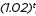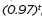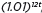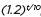# MAFS.912.F-IF.3.8

Write a function defined by an expression in different but equivalent forms to reveal and explain different properties of the function.
1. Use the process of factoring and completing the square in a quadratic function to show zeros, extreme values, and symmetry of the graph, and interpret these in terms of a context.
2. Use the properties of exponents to interpret expressions for exponential functions. For example, identify percent rate of change in functions such as y =, y =, y =, y =, and classify them as representing exponential growth or decay.
General Information
Subject Area: Mathematics
Domain-Subdomain: Functions: Interpreting Functions
Cluster: Level 2: Basic Application of Skills & Concepts
Cluster: Analyze functions using different representations. (Algebra 1 - Supporting Cluster) (Algebra 2 - Supporting Cluster) -

Clusters should not be sorted from Major to Supporting and then taught in that order. To do so would strip the coherence of the mathematical ideas and miss the opportunity to enhance the major work of the grade with the supporting clusters.

Date of Last Rating: 02/14
Status: State Board Approved
Assessed: Yes
Test Item Specifications
Also assesses:

MAFS.912.A-APR.2.3

MAFS.912.F-IF.3.7

• Assessment Limits :
For F-IF.3.7, items are limited to linear, quadratic, and exponential
functions.

For A-APR.2.3, the leading coefficient should be an integer and the
polynomial’s degree is restricted to 3 or 4. The polynomial function
should not have a zero with multiplicity. The polynomial should be
given in factored form.

For F-IF.3.8a, items that require the student to transform a quadratic
equation to vertex form, b/a must be an even integer.

For F-IF.3.7e and F-IF.3.8b, exponential functions are limited to simple
exponential growth and decay functions and to exponential functions
with one translation. Base e should not be used.

For F-IF.3.8, items may specify a required form using an equation or using common terminology such as standard form. In items that require the student to interpret the vertex or a zero of a quadratic function within a real-world context, the student should interpret both the x-value and the y-value.

For F-IF.3.7a, quadratic functions that are given in the form y = ax² + bx + c, a, b, and c must be integers. Quadratic functions given in vertex form y = a(x – h)² + k, a, h, and k must be integers. Quadratic functions given in other forms should be able to be rewritten and adhere to one of the two previous forms.

• Calculator :

Neutral

• Clarification :
Students will identify zeros, extreme values, and symmetry of a

Students will classify the exponential function as exponential growth
or decay by examining the base, and students will give the rate of
growth or decay.

Students will use the properties of exponents to interpret exponential
expressions in a real-world context.

Students will write an exponential function defined by an expression
in different but equivalent forms to reveal and explain different
properties of the function, and students will determine which form of
the function is the most appropriate for interpretation for a realworld context.

Students will find the zeros of a polynomial function when the
polynomial is in factored form.

Students will create a rough graph of a polynomial function in
factored form by examining the zeros of the function.

Students will use the x-intercepts of a polynomial function and end
behavior to graph the function.

Students will identify the x- and y-intercepts and the slope of the
graph of a linear function.

Students will identify zeros, extreme values, and symmetry of the

Students will identify intercepts and end behavior for an exponential
function.

Students will graph a linear function using key features.

Students will graph a quadratic function using key features.

Students will graph an exponential function using key features.

Students will identify and interpret key features of a graph within the
real-world context that the function represents.

• Stimulus Attributes :
Items may require the student to identify a correct graph.

Items may be set in a mathematical or real-world context.

For F-IF.3.8, items must use function notation.

For F-IF.3.7, items may use an equation or a function.

Items should not require the student to complete a sign chart for a
polynomial.

• Response Attributes :
For F-IF.3.7, items may require the student to apply the basic
modeling cycle.

Items may require the student to choose an appropriate level of
accuracy.

Items may require the student to choose and interpret the scale in a
graph.

Items may require the student to choose and interpret units.

Items may require the student to provide the answer in a specific
form.

Responses with square roots should require the student to rewrite
the square root so that the radicand has no square factors.

Sample Test Items (2)
• Test Item #: Sample Item 1
• Question:

A bird drops a stick from the top of Miami Tower. The height of the stick after x seconds is given by

f(x)=625-16x²

Select all the correct interpretations of the coordinates of the point at the maximum of the function f(x).

• Difficulty: N/A
• Type: MS: Multiselect

• Test Item #: Sample Item 2
• Question:

A bird drops a stick from the top of Miami Tower. The height of the stick after x seconds is given by

f(x)= 625-16x².

What is the maximum value of f(x)?

• Difficulty: N/A
• Type: EE: Equation Editor

## Related Courses

This benchmark is part of these courses.
1200310: Algebra 1 (Specifically in versions: 2014 - 2015, 2015 - 2022 (current), 2022 and beyond)
1200320: Algebra 1 Honors (Specifically in versions: 2014 - 2015, 2015 - 2022 (current), 2022 and beyond)
1200330: Algebra 2 (Specifically in versions: 2014 - 2015, 2015 - 2022 (current), 2022 and beyond)
1200340: Algebra 2 Honors (Specifically in versions: 2014 - 2015, 2015 - 2022 (current), 2022 and beyond)
1200380: Algebra 1-B (Specifically in versions: 2014 - 2015, 2015 - 2022 (current), 2022 and beyond)
1200400: Foundational Skills in Mathematics 9-12 (Specifically in versions: 2014 - 2015, 2015 - 2022 (current), 2022 and beyond)
1207310: Liberal Arts Mathematics (Specifically in versions: 2014 - 2015, 2015 - 2022 (course terminated))
1298310: Advanced Topics in Mathematics (formerly 129830A) (Specifically in versions: 2014 - 2015, 2015 - 2022 (course terminated))
1200500: Advanced Algebra with Financial Applications (Specifically in versions: 2014 - 2015 (course terminated))
1200410: Mathematics for College Success (Specifically in versions: 2014 - 2015, 2015 - 2022 (course terminated))
1200700: Mathematics for College Algebra (Specifically in versions: 2014 - 2015, 2015 - 2022 (course terminated))
7912090: Access Algebra 1B (Specifically in versions: 2014 - 2015, 2015 - 2018, 2018 - 2019, 2019 - 2022 (current), 2022 and beyond)
1200315: Algebra 1 for Credit Recovery (Specifically in versions: 2014 - 2015, 2015 - 2022 (current), 2022 and beyond)
1200335: Algebra 2 for Credit Recovery (Specifically in versions: 2014 - 2015, 2015 - 2019 (course terminated))
1200385: Algebra 1-B for Credit Recovery (Specifically in versions: 2014 - 2015, 2015 - 2022 (current), 2022 and beyond)
1201315: Analysis of Functions Honors (Specifically in versions: 2014 - 2015, 2015 - 2022 (course terminated))
7912075: Access Algebra 1 (Specifically in versions: 2014 - 2015, 2015 - 2018, 2018 - 2019, 2019 - 2022 (current), 2022 and beyond)
7912095: Access Algebra 2 (Specifically in versions: 2016 - 2018, 2018 - 2019, 2019 - 2022 (current), 2022 and beyond)
1200387: Mathematics for Data and Financial Literacy (Specifically in versions: 2016 - 2022 (current), 2022 and beyond)

## Related Access Points

Alternate version of this benchmark for students with significant cognitive disabilities.
MAFS.912.F-IF.3.AP.8a: Write or select an equivalent form of a function [e.g., y = mx + b, f(x) = y, y – y1 = m(x – x1), Ax + By = C].
MAFS.912.F-IF.3.AP.8b: Describe the properties of a function (e.g., rate of change, maximum, minimum, etc.).

## Related Resources

Vetted resources educators can use to teach the concepts and skills in this benchmark.

## Assessments

Sample 4 - High School Algebra 2 State Interim Assessment:

This is a State Interim Assessment for 9th-12th grade.

Type: Assessment

Sample 3 - High School Algebra 1 State Interim Assessment:

This is a State Interim Assessment for 9th-12th grades.

Type: Assessment

Sample 2 - High School Algebra 1 State Interim Assessment:

This is a State Interim Assessment for 9th-12th grades.

Type: Assessment

## Formative Assessments

Exponential Functions - 2:

Students are asked to identify the percent rate of change of a given exponential function.

Type: Formative Assessment

Exponential Functions - 1:

Students are asked to identify the percent rate of change of a given exponential function.

Type: Formative Assessment

A Home for Fido:

Students are asked to rewrite a quadratic function in an equivalent form by completing the square and to use this form to identify the vertex of the graph and explain its meaning in context.

Type: Formative Assessment

Launch From a Hill:

Students are asked to factor and find the zeros of a polynomial function given in context.

Type: Formative Assessment

## Lesson Plans

The Towers of Hanoi: Experiential Recursive Thinking:

This lesson is about the Towers of Hanoi problem, a classic famous problem involving recursive thinking to reduce what appears to be a very large and difficult problem into a series of simpler ones.  The learning objective is for students to begin to understand recursive logic and thinking, relevant to computer scientists, mathematicians and engineers.   The lesson is experiential, in that each student will be working with her/his own Towers of Hanoi manipulative, inexpensively obtained.  There is no formal prerequisite, although some familiarity with set theory and functions is helpful.  The last three sections of the lesson involve some more formal concepts with recursive equations and proof by induction, so the students who work on those sections should probably be level 11 or 12 in a K-12 educational system.  The lesson has a Stop Point for 50-minute classes, followed by three more segments that may require a half to full additional class time.  So the teacher may use only those segments up to the Stop Point, or if two class sessions are to be devoted to the lesson, the entire set of segments.  Supplies are modest, and may be a set of coins or some washers from a hardware store to assemble small piles of disks in front of each student, each set of disks representing a Towers of Hanoi manipulative.  Or the students may assemble before the class a more complete Towers of Hanoi at home, as demonstrated in the video.  The classroom activities involve attempting to solve with hand and mind the Towers of Hanoi problem and discussing with fellow students patterns in the process and strategies for solution.

Type: Lesson Plan

Functions and Everyday Situations:

This lesson unit is intended to help you assess how well students are able to articulate verbally the relationships between variables arising in everyday contexts, translate between everyday situations and sketch graphs of relationships between variables, interpret algebraic functions in terms of the contexts in which they arise and reflect on the domains of everyday functions and in particular whether they should be discrete or continuous.

Type: Lesson Plan

This lesson unit is intended to help you assess how well students are able to understand what the different algebraic forms of a quadratic function reveal about the properties of its graphical representation. In particular, the lesson will help you identify and help students who have the following difficulties in understanding how the factored form of the function can identify a graph's roots, how the completed square form of the function can identify a graph's maximum or minimum point, and how the standard form of the function can identify a graph's intercept.

Type: Lesson Plan

Exponential Graphing Using Technology:

This lesson is teacher/student directed for discovering and translating exponential functions using a graphing app. The lesson focuses on the translations from a parent graph and how changing the coefficient, base and exponent values relate to the transformation.

Type: Lesson Plan

## Perspectives Video: Expert

Using Mathematics to Optimize Wing Design:

Nick Moore discusses his research behind optimizing wing design using inspiration from animals and how they swim and fly.

Type: Perspectives Video: Expert

Which Function?:

The task addresses knowledge related to interpreting forms of functions derived by factoring or completing the square. It requires students to pay special attention to the information provided by the way the equation is represented as well as the sign of the leading coefficient, which is not written out explicitly, and then to connect this information to the important features of the graph.

Springboard Dive:

The problem presents a context where a quadratic function arises. Careful analysis, including graphing of the function, is closely related to the context. The student will gain valuable experience applying the quadratic formula and the exercise also gives a possible implementation of completing the square.

## Tutorial

Power of a Power Property:

This tutorial demonstrates how to use the power of a power property with both numerals and variables.

Type: Tutorial

## Unit/Lesson Sequence

Sample Algebra 1 Curriculum Plan Using CMAP:

This sample Algebra 1 CMAP is a fully customizable resource and curriculum-planning tool that provides a framework for the Algebra 1 Course. The units and standards are customizable and the CMAP allows instructors to add lessons, worksheets, and other resources as needed. This CMAP also includes rows that automatically filter and display Math Formative Assessments System tasks, E-Learning Original Student Tutorials and Perspectives Videos that are aligned to the standards, available on CPALMS.

Learn more about the sample Algebra 1 CMAP, its features and customizability by watching the following video:

### Using this CMAP

To view an introduction on the CMAP tool, please .

To view the CMAP, click on the "Open Resource Page" button above; be sure you are logged in to your iCPALMS account.

To use this CMAP, click on the "Clone" button once the CMAP opens in the "Open Resource Page." Once the CMAP is cloned, you will be able to see it as a class inside your iCPALMS My Planner (CMAPs) app.

To access your My Planner App and the cloned CMAP, click on the iCPALMS tab in the top menu.

All CMAP tutorials can be found within the iCPALMS Planner App or at the following URL: http://www.cpalms.org/support/tutorials_and_informational_videos.aspx

Type: Unit/Lesson Sequence

## Video/Audio/Animations

Solving Quadratic Equations using Square Roots:

This video will demonstrate how to solve a quadratic equation using square roots.

Type: Video/Audio/Animation

This video gives a more in-depth look at graphing quadratic functions than previously offered in Quadratic Functions 1.

Type: Video/Audio/Animation

## Worksheet

This worksheet gives students one place to show all transformations (reflections, vertical stretches/compressions, and translations) for the quadratic function. The worksheet also has a place for domain and range for each transformation.

Type: Worksheet

## MFAS Formative Assessments

A Home for Fido:

Students are asked to rewrite a quadratic function in an equivalent form by completing the square and to use this form to identify the vertex of the graph and explain its meaning in context.

Exponential Functions - 1:

Students are asked to identify the percent rate of change of a given exponential function.

Exponential Functions - 2:

Students are asked to identify the percent rate of change of a given exponential function.

Launch From a Hill:

Students are asked to factor and find the zeros of a polynomial function given in context.

## Student Resources

Vetted resources students can use to learn the concepts and skills in this benchmark.

Which Function?:

The task addresses knowledge related to interpreting forms of functions derived by factoring or completing the square. It requires students to pay special attention to the information provided by the way the equation is represented as well as the sign of the leading coefficient, which is not written out explicitly, and then to connect this information to the important features of the graph.

Springboard Dive:

The problem presents a context where a quadratic function arises. Careful analysis, including graphing of the function, is closely related to the context. The student will gain valuable experience applying the quadratic formula and the exercise also gives a possible implementation of completing the square.

## Tutorial

Power of a Power Property:

This tutorial demonstrates how to use the power of a power property with both numerals and variables.

Type: Tutorial

## Video/Audio/Animations

Solving Quadratic Equations using Square Roots:

This video will demonstrate how to solve a quadratic equation using square roots.

Type: Video/Audio/Animation

This video gives a more in-depth look at graphing quadratic functions than previously offered in Quadratic Functions 1.

Type: Video/Audio/Animation

## Parent Resources

Vetted resources caregivers can use to help students learn the concepts and skills in this benchmark.

Which Function?:

The task addresses knowledge related to interpreting forms of functions derived by factoring or completing the square. It requires students to pay special attention to the information provided by the way the equation is represented as well as the sign of the leading coefficient, which is not written out explicitly, and then to connect this information to the important features of the graph.

Springboard Dive:

The problem presents a context where a quadratic function arises. Careful analysis, including graphing of the function, is closely related to the context. The student will gain valuable experience applying the quadratic formula and the exercise also gives a possible implementation of completing the square.